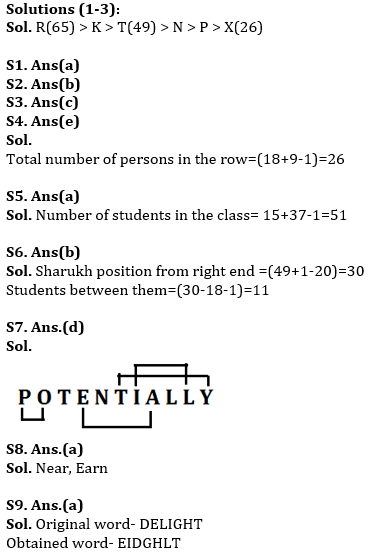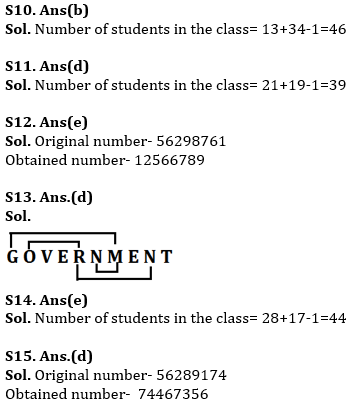Latest Banking jobs   »   Reasoning Ability Quiz For IBPS RRB...

# Reasoning Ability Quiz For IBPS RRB PO, Clerk Prelims 2021- 18th June

Directions (1-3): Study the following information carefully and answer the given questions.
Amongst Six persons, N, R, T, K, X and P, each has different Age. P’s age is not an even number. N age is more than only X and P. K age is less than only one person. R age is 65 yr and T age is 49 yr. Youngest person age is 26.

Q1. Who among the following is youngest person?
(a) X
(b) P
(c) K
(d) T
(e) R

Q2. What is possible age of K?
(a) 66yr
(b) 60yr
(c) 49yr
(d) 45yr
(e) None of these

Q3. If N’s age is 37yr, then what may be the age of P?
(a) 36
(b) 38
(c) 31
(d) 26
(e) Can’t be determined

Q4. Ranveer is 18th from the left end of a row and Deepika is 15th from the right end of row. If they interchanged their positions then Deepika ranks become 9 from right end. Find total number of persons in the row?
(a) 27
(b) 30
(c) 28
(d) 31
(e) None of these

Q5.Daniel ranked 15th from the top and 37th from the bottom in a class. How many students are there in the class?
(a) 51
(b) 50
(c) 52
(d) 49
(e) 48

Q6. Sharukh is 20th from the left end of a row of 49 students and Kajol is 18th from the right end in the same row. How many students are there between them in the row?
(a) 13
(b) 11
(c) 10
(d) 15
(e) 12

Q7. How many pairs of letters are there in the word “POTENTIALLY” each of which have as many letters between them in the word as they have between them in the English alphabetical series?
(a) One
(b) Two
(c) Three
(d) Four
(e) More than Four

Q8. How many words can be formed from the 1st, 5th, 8th and 9th letter of a word ‘ASTONOMERS’ by using each letter once in the word?
(a) two
(b) one
(c) none
(d) three
(e) more than three

Q9. If all the letters in the word DELIGHT are arranged in alphabetical order from left to right in such a way that vowels are arranged first followed by consonants, then how many letters are there in between D and L after the arrangement?
(a) two
(b) one
(c) none
(d) three
(e) four

Q10. Kamalpreet ranked 13th from the top and 34th from the bottom in a class. How many students are there in the class?
(a) 47
(b) 46
(c) 39
(d) 49
(e) 45

Q11. Sourav ranked 21th from the top and 19th from the bottom in a class. How many students are there in the class?
(a) 4o
(b) 41
(c) 38
(d) 39
(e) None of these

Q12. If in the number 56298761, all digits are arranged in increasing order from left to right then how many digit remain same place in the new arrangement?
(a) Three
(b) Four
(c) none
(d) Two
(e) One

Q13. How many pairs of letters are there in the word “Government” each of which have as many letters between them in the word as they have between them in the English alphabetical series?
(a) Three
(b) One
(c) Two
(d) More than three
(e) None

Q14. Kamal ranked 28th from the top and 17th from the bottom in a class. How many students are there in the class?
(a) 40
(b) 38
(c) 45
(d) 43
(e) 44

Q15. If in the number 56289174, 2 is subtracted to each of the digits which is greater than and equal to 6 and 2 is added from each of the digit which is less than 6 then how many digits are repeating in the number thus formed?
(a) two
(b) one
(c) none
(d) three
(e) four

Practice More Questions of Reasoning for Competitive Exams:

###### Study Plan for IBPS RRB PO/Clerk Prelims 2021

Solutions